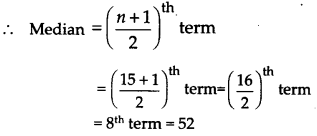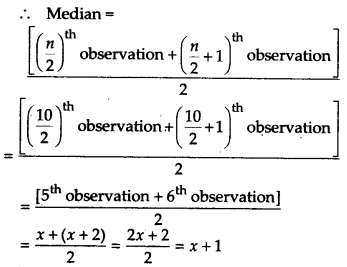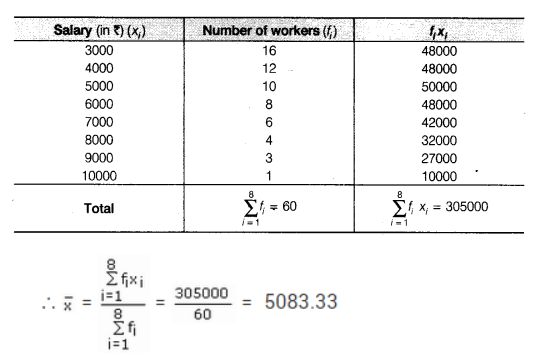NCERT Solutions for Class 9 Maths Chapter 14 Statistics Ex 14.4

# NCERT Solutions for Class 9 Maths Chapter 14 Statistics Ex 14.4

## NCERT Solutions for Class 9 Maths Chapter 14 Statistics Ex 14.4

NCERT Solutions for Class 9 Maths Chapter 14 Statistics Ex 14.4 are the part of NCERT Solutions for Class 9 Maths. Here you can find the NCERT Solutions for Chapter 14 Statistics Ex 14.4.

Ex 14.4 Class 9 Maths Question 1.
The following number of goals were scored by a team in a series of 10 matches:
2, 3, 4, 5, 0, 1, 3, 3, 4, 3
Find the mean, median and mode of these scores.

To find the median:
Arranging the given data in ascending order, we have

0, 1, 2, 3, 3, 3, 3, 4, 4, 5
n = 10, an even number

Thus, median = 3

To find the mode:
In the given data, the observation 3 occurs 4 times, i.e., the maximum number of times.
Thus, mode = 3

Ex 14.4 Class 9 Maths Question 2.
In a mathematics test given to 15 students, the following marks (out of 100) are recorded:
41, 39, 48, 52, 46, 62, 54, 40, 96, 52, 98, 40, 42, 52, 60
Find the mean, median and mode of this data.

To find the median:
Arranging the given data in ascending order, we have
39, 40, 40, 41, 42, 46, 48, 52, 52, 52, 54, 60, 62, 96, 98
n = 15 (an odd number)Thus, median = 52

To find the mode:
In the given data, the observation 52 occurs 3 times, i.e., the maximum number of times.
Thus, mode = 52

Ex 14.4 Class 9 Maths Question 3.
The following observations have been arranged in ascending order. If the median of the data is 63, find the value of x.
29, 32, 48, 50, x, x + 2, 72, 78, 84, 95

Solution:
Here, the given observations are in ascending order.

Since, n = 10 (an even number)Since, median = 63 [Given]
x + 1 = 63 x = 63 – 1 = 62
Thus, the required value of x is 62.

Ex 14.4 Class 9 Maths Question 4.
Find the mode of 14, 25, 14, 28, 18, 17, 18, 14, 23, 22, 14 and 18.

Solution:
Arranging the given data in ascending order, we have

14, 14, 14, 14, 17, 18, 18, 18, 22, 23 25, 28
Since the observation 14 is occurring the maximum number of times (i.e., 4 times).
Mode of the given data = 14

Ex 14.4 Class 9 Maths Question 5.
Find the mean salary of 60 workers of a factory from the following table:

Solution:Thus, the required mean salary = Rs. 5083.33

Ex 14.4 Class 9 Maths Question 6.
Give one example of a situation in which
(i) the mean is an appropriate measure of central tendency.
(ii) the mean is not an appropriate measure of central tendency but the median is an appropriate measure of central tendency.

Solution:
(i) Mean height of the students of a class.

(ii) Median weight of a pencil, a book, a rubber band, a lunchbox and a chair.

You can also like these:

NCERT Solutions for Maths Class 10

NCERT Solutions for Maths Class 12